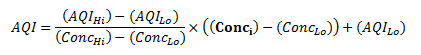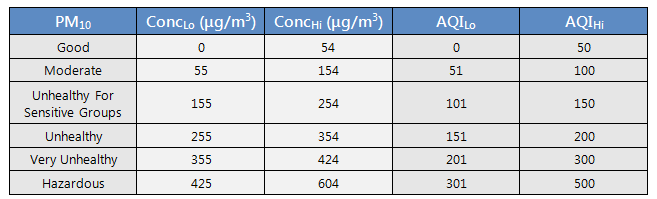# The AQI Equation

## AQI EquationWhere
Conci = Input concentration for a given pollutant
ConcLo = The concentration breakpoint that is less than or equal to Conci
ConcHi = The concentration breakpoint that is greater than or equal to Conci
AQILo = The AQI value corresponding to Conci
AQIHi = The AQI value corresponding to Conci

## Rounding vs. Truncating

For all AQI calculations, the concentrations are truncated to the nearest .001 ppm (1 ppb) for O3, 0.1 μg/m3 for PM2.5, and 1 μg/m3 for PM10. This truncated concentration is then used as the input (Conci) in the AQI equation above. The resulting AQI is rounded to the nearest whole number.

## AQI and Concentration Breakpoints by Pollutant (2015)AQI values above 500 are considered Beyond the AQI. Follow recommendations for the Hazardous category. Additional information on reducing exposure to extremely high levels of particle pollution is available here.